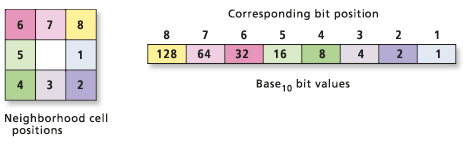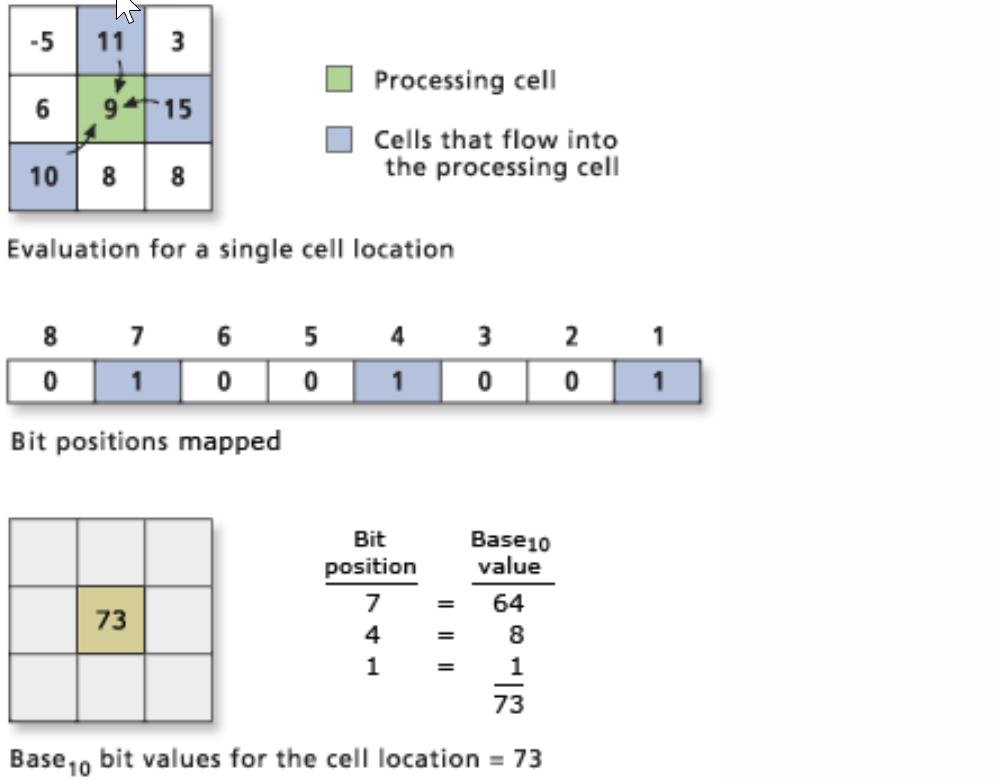# How to identify the highest and lowest pixels within a defined neighborhood area of a central pixel

5940
28
05-31-2017 04:13 PM

Hi,

I am using the 'range' statistics type within the 'focal statistics' tool. For each central pixel ArcGIS calculates the difference between the highest and lowest values in the defined neighborhood area and assigns it to the central pixel. What I am now looking for is a way to identify the highest and lowest pixels within that defined neighborhood area.

Any help is much appreciated.

Regards,

Fidel

28 Replies
byMVP Esteemed Contributor

focal statistics minimum and focal statistics max  plus all the other tools in the focal statistics tools in ArcToolbox will cover all combinations and permutations of gleaning other information

Hi Dan,

Yes, but what I am trying to find out is to actually locate the max and min pixels. The focal statistics tools assign the results of the operation to the central pixel and do not give the actual location of the max/min pixels as an output.

Regards,

Fidel

byEsri Esteemed Contributor

You can use Focal Flow—Help | ArcGIS Desktop  to determine the location of the lowest value. To determine the maximum value, invert your raster (highest value - original raster) and run again.

byMVP Esteemed Contributor

so if you are using a focalmin, for example, it will determine the minimum value and assign it to the middle value.  Try subtracting the original raster from that raster and values of 0 should identify the cell location where the minimum occurred.

byEsri Esteemed Contributor

If the OP needs to identify the lowest and highest position in a 3x3 window for each pixel I think it will need the Focal Flow to obtain that.  Each pixel will obtain a value to points to the relative position where the highest or lowest pixel is located.byMVP Esteemed Contributor

From the link on focal flow or even flow direction, they do use the surrounding cells in their calculation.  In the case of focal flow, the cells that contribute the core cell. they don't tell you the exact location of the cells represent the minimum.  In the example below, we should get a value of -5 for the minimum, 15 for the maximum and 20 for the range assigned to the core cell.  If we use the focal minimum raster and subtracted the input raster from it, we should get a 0 for the top left cell.  Values of 0 would be the minimum of one of the moving windows, assuming raster processing is from top left, then the value of 0 would correspond to core cells in standard row/column format.

From Focal FlowNow having said that, if the raster were exported to a numpy array, there are other ways of doing this even using rolling statistics or queries.

``aarray([[-5, 11,  3],       [ 6,  9, 15],       [10,  8,  8]])m = np.min(a, (0,1)) # along the row, column... returns -5np.argwhere(a == m)array([[0, 0]], dtype=int64) # row 0, column 0‍‍‍‍‍‍‍‍‍``
byMVP Esteemed Contributor

Here is how to locate each cell that is the highest or lowest value in its own 3x3 neighborhood. Not sure this is exactly what you want, but I thought I'd throw it out there.

The expressions below are formatted for the Raster Calculator tool. BTW, I used "" for neighborhood to use the default, NbrRectangle(3,3).

``# Cells that are the lowest of the 3x3 around that cellCon(FocalStatistics("in_grid", "", "MINIMUM") == "in_grid"), "in_grid")# Cells that are the highest of the 3x3 around that cellCon(FocalStatistics("in_grid", "", "MAXIMUM") == "in_grid", "in_grid")‍‍‍‍‍‍‍‍‍‍‍‍‍‍‍‍‍‍‍‍‍‍‍‍‍‍‍‍``

Thank you all for the help so far.

The suggested methods are unfortunately not doing what I want to do. This is mainly because the neighborhood zone is overlapping as the operation moves from one cell to another cell. The minimum cell for the first central cell will change its value to the minimum value for its own neighborhood as the operation moves from cell to cell. As a result subtracting the minimum raster from the original raster (or the method suggested by Curtis) will not give me the locations of all the minimum cells for each neighborhood zone.

Any more suggestions are appreciated.

Cheers

Fidel

byEsri Esteemed Contributor

Have you tried the Flow Direction tool yet? It will return the lowest value for each pixel in a 3x3 neighborhood.